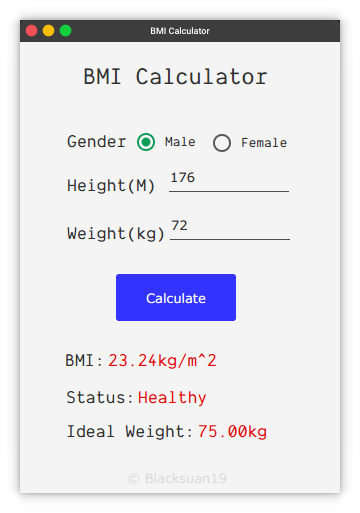## BMI Calculator#### Introduction:

A simple BMI calculator build in javaFX

#### Why:

another case of boredom

#### Features:

• calculate BMI
• Display current weight status
• calculate ideal weight
• GUI build with JFoenix Library

#### Calculations:

the standard BMI formula is known and can ve found easily, the hard part is calculating the idea body weight, which i used robinson’s formula (1989) for it.

#### Screenshots: